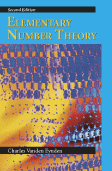278 pages, \$69.95 list
1-57766-445-0
978-1-57766-445-1
Instructor's Solutions Manual available
eBook availability
Elementary Number Theory
Second Edition
This practical and versatile text evolved from the author's years of teaching experience and the input of his students. Vanden Eynden strives to alleviate the anxiety that many students experience when approaching any proof-oriented area of mathematics, including number theory. His informal yet straightforward writing style explains the ideas behind the process of proof construction, showing that mathematicians develop theorems and proofs from trial and error and evolutionary improvement, not spontaneous insight. Furthermore, the book includes more computational problems than most other number theory texts to build students' familiarity and confidence with the theory behind the material. The author has devised the content, organization, and writing style so that information is accessible, students can gain self-confidence with respect to mathematics, and the book can be used in a wide range of courses—from those that emphasize history and type A problems to those that are proof oriented.
Reactions
"The book is excellent. There is a good mix of routine and more challenging problems. The exposition is very good, too. Students found the book very user-friendly." — Phillip Lestmann, Bryan College
0. What Is Number Theory?

1. Divisibility
The GCD and LCM / The Division Algorithm / The Euclidean Algorithm / Linear Combinations / Congruences / Mathematical Induction

2. Prime Numbers
Prime Factorization / The Fundamental Theorem of Arithmetic / The Importance of Unique Factorization / Prime Power Factorization / The Set of Primes is Infinite / A Formula for ?(n)

3. Numerical Functions
The Sum of the Divisors / Multiplicative Functions / Perfect Numbers / Mersenne and Fermat Numbers / The Euler Phi Function / The Moebius Inversion Formula

4. The Algebra of Congruence Classes
Solving Linear Congruences / The Chinese Remainder Theorem / The Theorems of Fermat and Euler / Primality Testing / Public-Key Cryptography

5. Congruences of Higher Degree
Polynomial Congruences / Congruences with Prime Power Moduli / Quadratic Residues / Quadratic Reciprocity / Flipping a Coin over the Telephone

6. The Number Theory of the Reals
Rational and Irrational Numbers / Finite Continued Fractions / Infinite Continued Fractions / Decimal Representation / Lagrange's Theorem and Primitive Roots

7. Diophantine Equations
Pythagorean Triples / Sums of Two Squares / Sums of Four Squares / Sums of Fourth Powers / Pell's Equation